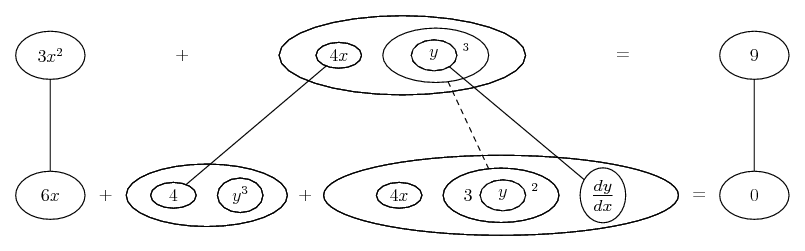# Thread: Implicit Differentiation #2

1. ## Implicit Differentiation #2

find dy/dx

3x^2+4xy^3=9
6x(dy/dx)+4y^3+4x3y^2(dy/dx)=0
dy/dx(6x+4x3y^2)=-4y^3

dy/dx=(-4y^3)/(12xy^2+6x)
dy/dx=(-4y^3)/6x(2y^2+1)

can someone check to see if this is correct?

2.Originally Posted by skeske1234find dy/dx

3x^2+4xy^3=9
6x(dy/dx)+4y^3+4x3y^2(dy/dx)=0
dy/dx(6x+4x3y^2)=-4y^3

dy/dx=(-4y^3)/(12xy^2+6x)
dy/dx=(-4y^3)/6x(2y^2+1)

can someone check to see if this is correct?
sorry, it's not.

$\displaystyle \frac{d}{dx} (3x^2 + 4xy^3 = 9)$

$\displaystyle 6x + 4x \cdot 3y^2 \frac{dy}{dx} + y^3 \cdot 4 = 0$

$\displaystyle 12xy^2 \frac{dy}{dx} = -(6x + 4y^3)$

$\displaystyle \frac{dy}{dx} = -\frac{3x + 2y^3}{6xy^2}$

3. Just in case a picture helps...Don't integrate - balloontegrate!
Balloon Calculus: worked examples from past papers

#### Search Tags

differentiation, implicit